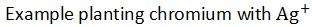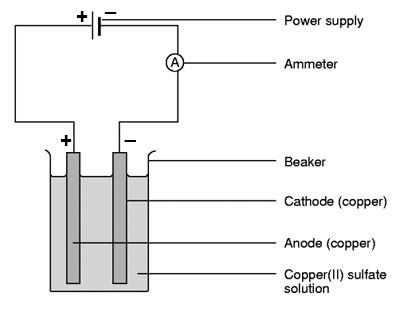CHEMISTRY O LEVEL(FORM THREE) – IONIC THEORY AND ELECTROLYSIS ELECTROLYSIS.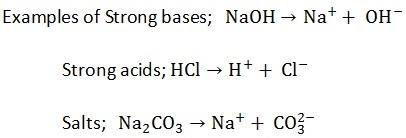Written by

IONIC THEORY AND ELECTROLYSIS

ELECTROLYSIS.
Definition: is the decomposition of electrolytes by the passage of an electric current through it.
Electrolyte:
This is the substance which either in solution or in molten state conducts electric current to undergo decomposition due to its passage.
For example; H2SO4 – Sulphuric acid
HNO3 – Nitric acid
NaCl – Sodium chloride
Salts, acids and bases
A non – electrolyte
This is a substance which either in solution or molten state doesn’t conduct an electric current
Example Sugar, ethanol, urea etc.

Types of electrolytes
There are two types of electrolytes
(a) Strong electrolyte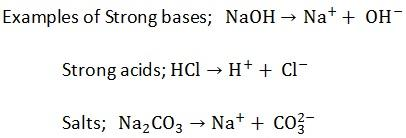These are electrolytes which ionize completely in solution example H2SO4, HCl, NaCl, and NaOH
b) Weak electrolytes
These are electrolytes which ionize partially in solution. Examples are,
Weak acids such as CH3COOH, H2CO3
Water
Weak bases such NH3 solution or NH4OH
MECHANISM OF ELECTROLYSIS
When an electrolyte is dissolved in water it dissociates to form ions thus the aqueous solution of electrolyte contains positive and negative ions
Example strong electrolyte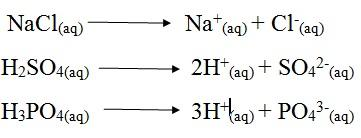Weak electrolytes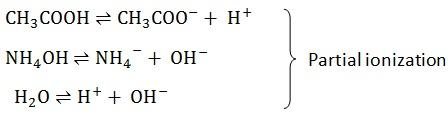IONS:
Ion is a charged particle
It’s either positively charged or negatively charged
a) Anions – are negatively charged ions
b) Cations – are positively charged ions
THE ELECTRODES:
These are the two poles of metals rods or carbon by which the electrons leave or enter the electrolyte
Types of electrodes
a) The Anode (+)
This is the positive electrode by which the electrons leave the electrolyte
b)The cathode ()
This is the negative electrode by which electrons enter the electrolyte
When an electric current passes through an electrolyte, the ions move to the oppositely charged electrodes.
The Cations (positive ions) move to cathode
The anions (negative ions) move to anode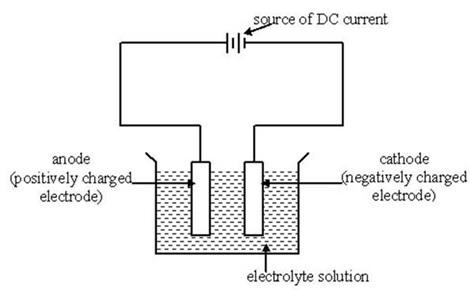ELECTRODE REACTIONS
1. Anode reaction
Anions carry negative charges due to extra electrons they have.
On arrival at the surface of anode they surrender the extra electron. By so doing they become discharged.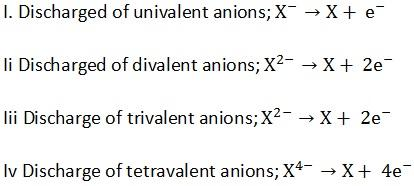Any process involving the loss of electrons is called oxidation. In electrolysis the reaction at the anode is called oxidation.
Therefore,another definition of oxidation;
This is the process whereby the substance looses electrons.
2. Cathode reaction
Cations have positive charges because they have deficiency of electrons. On arrival at the surface of cathode, they receive electrons from the cathode.
By so doing they become discharged

Example; i) Univalent cations discharged as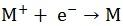ii) Divalent Cations discharge as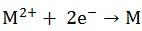iii) Trivalent Cations discharge as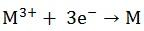Any process involving the gain of electrons is called reduction. The cathode reaction is reduction process.
Therefore, reduction is the process whereby the substance gains electrons.
PREFERENTIAL DISCHARGE OF IONS:
When two or more ions of similar charge are present under similar conditions in a solution.
2g H+ and Na+ or OH and SO42-, one of them is preferentially selected for discharge
The selection of ion to discharge depends on the following factors;
a) Position of the metal or radical in the electrochemical series
b) Concentration
c) Nature of the electrode
A POSITION OF THE METAL/GROUP IN ELECTROCHEMICAL SERIES
The electrochemical series is an arrangement of metal ions in the order which can be reduced (discharged). Those which can be reduced (discharged) with difficult are placed at the top of the series example K+, Ca2+ and Na+ may accept electrons with great difficulty. Ag+ and Cu2+ can accept electrons readily/ easily.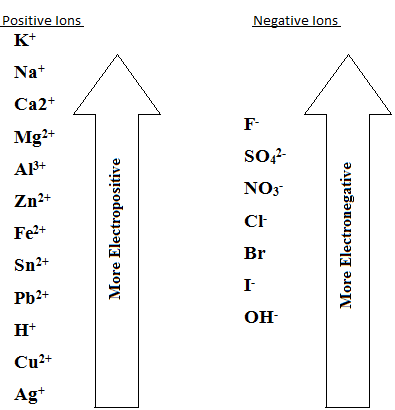The ion tower in electrochemical series is discharged in preference, to those above it
Example; electrolysis of dilute hydrochloric acid using platinum electrode ions present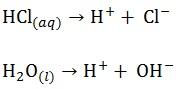Cathode reaction
H+ will migrate to the cathode and be dischargedH2 gas will evolve at the cathode
Anode reaction
Cl and OH will migrate to and OH will be preferentially discharged because it is lower than Clin electrochemical series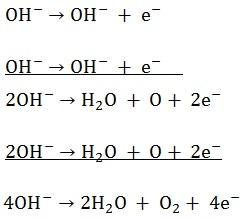O2 gas will evolve at the anode
Conclusion: The electrolyte concentration will be increasing as the elements of water are the ones coming out as gases
ELECTROLYSIS OF DILUTE SULPHURIC ACID (using platinum electrode)
ELECTOLYSIS OF WATER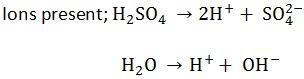Anode reaction
SO42- and OHwill migrate to the anode and OHwill be preferentially selected to dischargeO2 (g) will evolve at the anode
Cathode reaction
H+ will migrate to the cathode and dischargeHydrogen will evolve at the cathode
Overall effect
The concentration of the electrolyte will increase because during electrolysis the elements of water comes out as gases
CONCENTRATION OF THE IONS
Increase in concentration of an ion(s) tends to promote its discharge
Example Electrolysis of concentrated hydrochloric acid.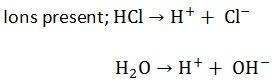Anode reaction
Cl and OHwill migrate to the anode and Cl will be discharged in preference to the OHbecause it’s available in higher concentration.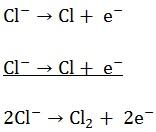Chlorine gas will evolve at the anode
Cathode reactionHydrogen gas will evolve at the cathode
Overall effect.
-Concentration of electrolyte will be decreasing because elements of HCl are moving out as gases.
NB: whenever a halogen (Cl, Br, I) is involved in competition for discharge, the concentration factor holds an upper hand especially chloride salts, salts of Halogens e.g. PbBr2
NATURE OF THE ELECTRODE
In some reactions of electrolysis the type of electrode used will determine the products of electrolysis.
Consider the following examples
1. a) Electrolysis of sodium chloride by using carbon.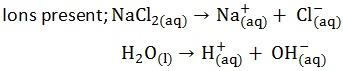At the cathode both Na+ and H+ will migrate to the cathode. H+ will be selected to discharge in the preference to Na+ due to Na+ position in electrochemical series
Cathode reactionAt the anode
Both Cl and OH will migrate to the anode and Cl will be discharged due to the concentration factorH2 (g) will evolve at the cathode and
Cl2 (g) will evolve at the anode
B) Electrolysis of sodium chloride by using mercury cathode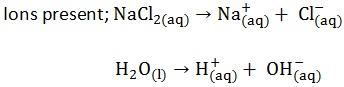At the cathode At the anode Both H+ and Na+ will move to the cathode of mercury. But it’s Na+ which will be discharged due to the nature of electrode. Both Cl– and OH– will move here and due to the concentration factor. Cl– will be discharged in the preference to OH–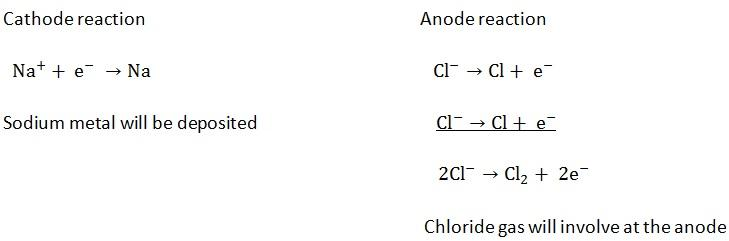2(a) Electrolysis of CuS042- by using Carbon / platinum electrode: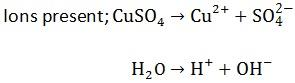At the cathode
Ions present are Cu2+ and H+
At cathode reaction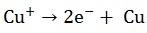Because Cu2+ is lower in e.c.s copper metal will be deposited at the cathode
At the anode.
Ions present are SO42- and OH
Anode reaction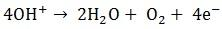Oxygen gas will be produced at the anode.
Overall effect
a) Reddish brown copper metal is deposited on cathode
b) O2 (g) is liberated at the anode
c) Concentration of CuSO4 decrease i.e. blue colour of CuSO4 turns to colourless since the copper II sulphate is converted to form sulphuric acid or the number of Cu2+ in the solution decreases
2(b) Electrolysis of CuSO4 using copper electrodesAt the cathode
Ions moved are Cu2+ and H+ cathode reaction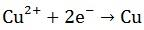Copper metal is produced at the cathode
At the anode
Ions moved here are SO42- and OH
Anode reaction
When copper anode is used there are 3 possibilities
I) Discharged of SO42-
ii) Discharge of OH
Iii) Conversion of Cu atoms to Cu2+
The conversion of Cu to Cu2+ occurs most readily
Anode reaction therefore is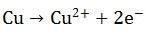Copper ions are released into the solution
Overall effect
a) Reddish brown copper metal is deposited at the cathode
b) Copper ions released into the solution from anode
c) Concentration of CUSO4 remains constant since the number of Cu2+ discharged at the cathode are replaced by the released Cu2+ from the anode. Hence no colour change.
d) Anode decreases in weight
This electrolysis is only the transfer of copper from anode to cathode.
LAWS OF ELECTROLYSIS;
A man called faraday put forward two laws expressing results of experiments of electrolysis. These laws they assert that the amount of an element liberated during electrolysis depends on
i) The time of passing the steady current
ii) The magnitude of the steady current
iii) The charge on the ion of the element
It states that;
“The mass of a substance liberated at or absorbed from an electrode during electrolysis is directly proportional to the quantity of electricity passed through the electrolyte”
If symbols are used the first law can be stated mathematically as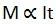Where;m = mass of the substance liberated
t = time in seconds
I = current in Amperes.
To replace the symbol of proportionality by an equal sign we must introduce a proportionality constant Z
m = zIt
But It = Q, where Q = quantity of electricity
m = ZQ
Z = is known as electrochemical equivalent of an element
Definition;
The electrochemical equivalent of a substance is the mass of a substance that can be liberated by passage of one coulomb
Its SI unit is g/c
Every element has its own value of electrochemical equivalent of an element
To calculate the value of Z of an element we use the formula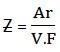Where; Ar = relative atomic mass of an element

V = valency of the element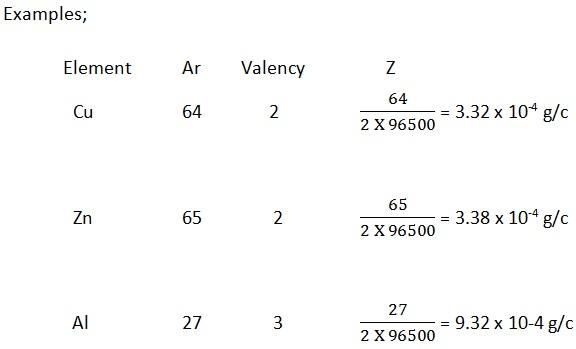NB:Faraday’s constant is the quantity of electric charge carried by electrons

(It is 96500 C) per mole
It states that
“When the same quantity of electricity is passed through solutions of different electrolytes, the amounts of elements deposited are proportional to the chemical equivalent of the element”
Chemical equivalent of the element is obtained by dividing it’s relative atomic mass by the charge of its ion
Example Chemical equivalent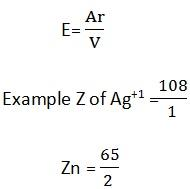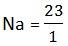Mathematically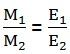MATHEMATICAL STATEMENT OF FARADAY’S 2ND LAW OF ELECTROLYSIS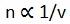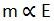Where v = charge of ion m = C.E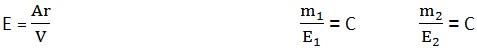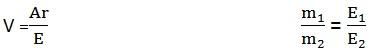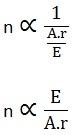n.Ar = C.E
m = C.E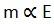QUESTION;
In an experiment, 1930c liberated 0.64g copper. When the same quantity of electricity was passed through a solution containing silver ions (Ag+), what amount of Ag was deposited. Explain the results
MAg =?
Mcu = 0.64g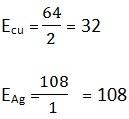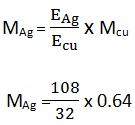MAg = 21.6g
Mass of silver is 21.6g
Mass of silver is large than of copper because silver has a small chemical equivalent than that of copper.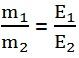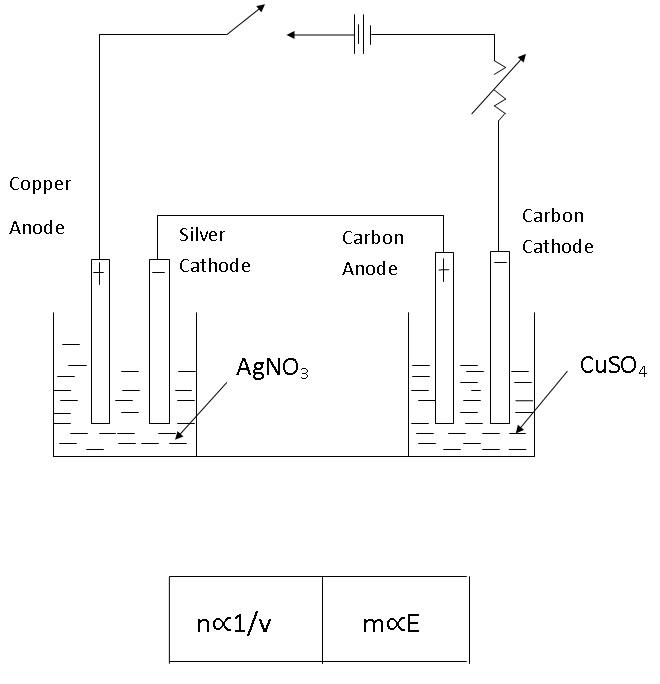QUESTIONS:
1. A silver voltmeter is connected in series with copper voltmeter. What weight of Ag will be deposited in one voltmeter while 0.16g of copper is deposited in the other?
2. The same current passes through solution of same concentration of PgNO3 and CuSO4 liberated 0.23 of Ag of the same current
Chemical equivalent of Cu = 3.18 and Ag = 108.
3. A current was passed through a voltammeter arranged in series. If the weight of copper on the electrode of cells no 1 was 1.27.Find the weight of Ag deposited on the cathode of cell number 2, if same quantity of electricity is passed through
Chemical equivalent of Ag = 108
Cu = 31.75
4. An electric current was passed in series through solutions of calcium chloride and CuSO4. Carbon electrodes were used in both electrolytes. If 2.5l of chlorine measured at S.T.P were produced, what volume of oxygen would also be produced? What mass of copper was produced?
Solution
1. Mcu = 0.16
MAg =?
Ecu = 64 x ½ = 32
EAg = 108/1 = 108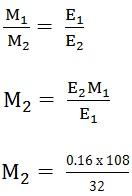MAg = 0.54g
1kg = 1000g

? = 0.54g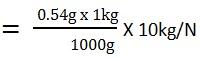WAg = 0.0054N.

2. MAg = 0.23g
Ecu = 31.8
EAg = 108
Mcu =?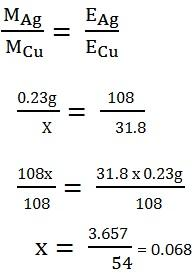MCu=0.068g.
3. MCu = 1.27g
MAg =?
EAg = 108
ECu=31.75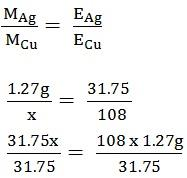X = 4.3g
MAg = 4.3g.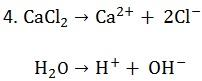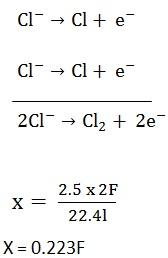X = 0.223F
Since same current is passed;
1. Volume of oxygen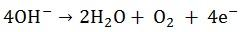4F = 22.4dm3
0.223F =?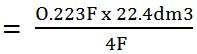= 1.25dm3
Mass of Cu produced?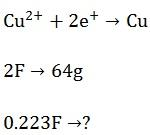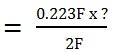= 7.14g
APPLICATION OF ELECTROLYSIS
1. PURIFICATION OF METALS
In the method blocks of the impure metal are made at the anode and sheets of extremely pure metal are made at the cathode
Example; Copper is industrially purified by the electrolysis of aqueous copper II sulphate using copper electrodes where anode is impure and cathode is pure.
Also Zn is purified by electrolysis of Zn sulphate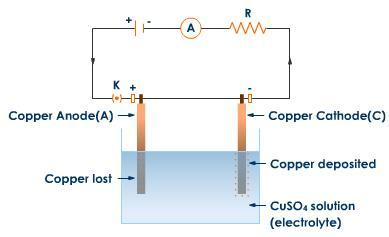2. MANUFACTURE (EXTRACTION) OF ELEMENTS
Very reactive elements are extracted by electrolysis of their molten chlorides e.g. Al, Mg, Na, K (Details in extraction of metals)
There is chemical combination
Example Au is less reactive this can be extracted without electrolysis is process
3. ELECTROPLATING
This is the process of covering the corroding material (metals) by the unreactive metals using electrolysis to prevent corrosion and improve their appearance.
The article to be plated is made the cathode and the anode is made of the planting material